# Activity 10.4 NCERT Class 10 Science, Light Reflection and Refraction

### Activity 10.4 NCERT Class 10 Science, chapter 10, Light Reflection and Refraction

###### Brief procedure:

Activity 10.4 asks us to make a ray diagram of objects placed at various positions in front of a concave mirror.

###### Rules:

There are simple rules to make a ray diagram. They are:

1. A ray of light parallel to the axis of the mirror reflects towards focal length.
2. Light passing through focal length will emerge parallel to the axis. This is inverse of the above rule.
3. A ray of light passing through the centre of the mirror (pole) return with the same path.
4. Light passing from the pole of the mirror reflects at the same angle.

Now let us see the ray diagram at various positions:

###### a. An object at infinity.

Here all light rays will be parallel to the axis of the mirror. So as per rule 1, they reflect toward the focal length.

Here the image will be real, highly diminished like a point and inverted.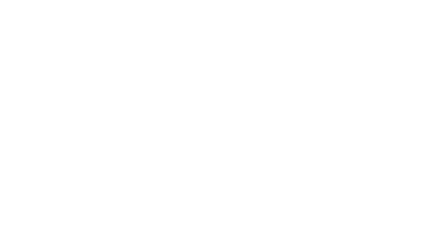An object at infinity from a concave mirror
###### b. Object beyond C

To make a ray diagram of this case we pass three rays. One, from the base of the object B to the principal axis. It returns on the same line. A second line, parallel to the principal axis from A. It reflects towards the focal point. You can take 3rd ray either toward the centre or towards the focal length. Result in both cases will be the same.

Imaged formed here is inverted, diminished and real.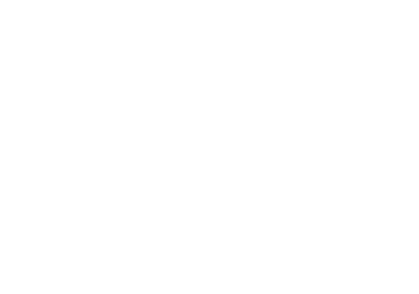An object beyond the centre of the concave mirror.
###### c. At c:

We apply the same process as of b. One ray from B to principal axis; One ray parallel to the axis; and another either towards the centre or focal length.

The image formed here is of the same size, inverted and real.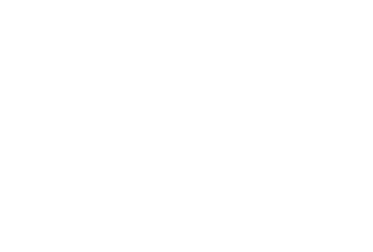An object at the centre of concave mirror
###### d. Between C and F:

Here also the image is real and inverted but magnified.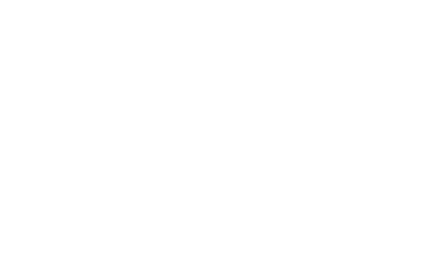An object between the centre and focal length of a concave mirror.
###### e. At F:

Here the image is real, inverted and highly magnified.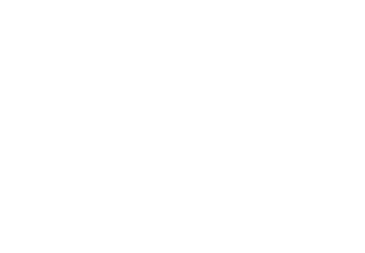An object at the focal point of a concave mirror.
###### f. Between P and F:

Here we apply the similar process, but here rays do not intersect to give us the idea of the position of the image. In such cases, we draw an imaginary line beyond the mirror. By doing this, we find that rays intersect behind the mirror at some distance.

Here the image is magnified, erect and unreal. We say unreal because we cannot place a paper and see the image. In case of real images, like above cases of a. to e., we can put a paper at the image position and see the imprint.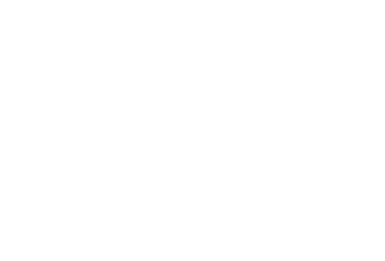An object between focal point and pole of the concave mirror.

Next: Formation of an image in a convex mirror. Activity 10.5.

### 2 thoughts on “Activity 10.4 NCERT Class 10 Science, Light Reflection and Refraction”

1.nice……………………………………

2.